# Complex number facts for kids

Kids Encyclopedia Facts
(Redirected from Complex numbers)

A complex number is a number, but is different from normal numbers in many ways. A complex number is made up using two numbers combined together. The first part is a real number. The second part of a complex number is an imaginary number. The most important imaginary number is called$i$, defined as a number that will be -1 when squared ("squared" means "multiplied by itself"). All the other imaginary numbers are$i$ multiplied by a real number, in the same way that all real numbers can be thought of as 1 multiplied by another number. Arithmetic functions such as, addition, subtraction, multiplication, and division can be used with complex numbers. They also follow commutative, associative and distributive properties, just like real numbers.

Complex numbers were invented to answer special equations that have exponents in them. These began to pose real problems for mathematicians. As a comparison, using negative numbers, it is possible to find the x in the equation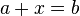$a + x = b$ for all real values of a and b.

With exponentiation, there is a problem. There is no real number that gives −1 when it is squared. In other words, −1 (or any other negative number) has no real square root. To solve this problem, mathematicians introduced an imaginary number called i. That imaginary number will give −1 when it is squared.

The first mathematicians to have thought of this were probably Gerolamo Cardano and Raffaele Bombelli. They lived in the 16th century. It was probably Leonhard Euler who introduced writing$\mathrm i$ for that number.

All complex numbers can be written as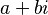$a + bi$ (or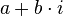$a + b \cdot i$ ), where a is called the real part of the number (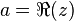$a = \Re (z)$ or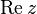$\operatorname{Re} z$), and b is called the imaginary part (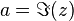$a = \Im (z)$ or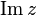$\operatorname{Im} z$). The complex number can also be written as a set (a, b). Both a and b are real numbers. Any real number can simply be written as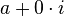$a + 0 \cdot i$ or as the set (a, 0).

The set of all complex numbers is usually written as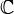$\mathbb{C}$.

## Operations over complex numbers

Addition, subtraction, multiplication, division as long as the divisor is not zero, and exponentiation (raising numbers to exponents) are all possible with complex numbers. Some other calculations are also possible with complex numbers.

The rule addition of complex numbers is pretty simple: let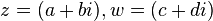$z = (a + bi), w = (c + di)$, then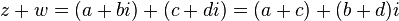$z + w = (a + bi) + (c + di) = (a + c) + (b + d)i$. Multiplication is a bit different: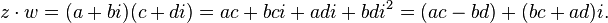$z \cdot w = (a + bi)(c + di) = ac + bci + adi + bdi^2 = (ac - bd) + (bc + ad)i.$

Another notable operation for complex numbers is conjugation. A complex conjugate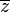$\overline{z}$ to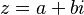$z = a + bi$ is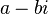$a - bi$. It is pretty simple, but is important for calculations, because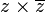$z \times \overline{z}$ belongs to the real numbers for all complex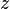$z$.

## Other forms of describing complex numbers

Complex numbers can be shown on a so-called complex plane. If you have a number$z = a + bi$, you can go to a point on the real axis and to b on the imaginary and draw a vector from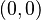$(0, 0)$ to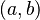$(a, b)$. You can calculate the length of this vector using the Pythagorean theorem and angle between the real axis and this vector, counting counterclockwise. The length of a vector for a number$z$ is called modulus (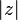$|z|$), and the angle is argument (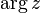$\arg z$).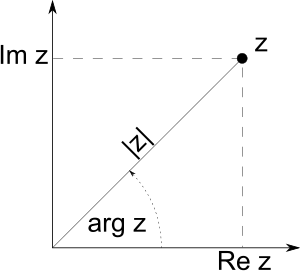A complex number can be visually shown as two numbers which form a vector on an Argand diagram, representing the complex plane.

This leads to the trigonometrical form of describing complex numbers: by the definitions of sine and cosine, for all$z$ stands that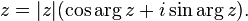$z = |z|(\cos \arg z + i \sin \arg z).$

This is closely connected to De Moivre's formula.

There exists even another form, called exponential.

## Conclusion

With the addition of complex numbers to math, every root of a polynomial with complex coefficients is a complex number. The successful addition of the complex numbers to math also help to open a path to the creation of another sorts of numbers that could resolve and help to explain many different problems, for example the: hypercomplex numbers, sedenion, hyperreal numbers, surreal numbers and many others. See types of numbers.Complex number Facts for Kids. Kiddle Encyclopedia.